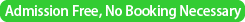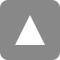﻿ Tutorial Lecture by Prof. Michael Mascagni (Supported by Research and Development Center for Data Assimilation, ISM) | 統計数理研究所

# Tutorial Lecture by Prof. Michael Mascagni (Supported by Research and Development Center for Data Assimilation, ISM)

Date&Time
December 19, 2016
10:00-12:00, 13:30-17:00 (with short intermissions)Place
Seminar room 2 @ The Institute of Statistical Mathematics
Speaker
Prof. Michael Mascagni
(Department of Computer Science, Florida State University, USA)
Title
An Introduction to Brownian Motion, Wiener Measure, and Partial Differential Equations
Abstract
This is a long introduction to how stochastic processes and partial differential equations are related. We begin with an introduction to Brownian motion, which is a Markoffian stochastic process. We give some of it's elementary properties and present the notion of expectation with respect to Brownian motion, and introduce Wiener measure. We then compute some Wiener integrals, and derive an explicit representation of Brownian motion. We then introduce the Feynman-Kac formulas for the probabilistic representation of partial differential equations. Time permitting, we explore some asymptotic results in action- and entropy-asymptotics.  We also introduce the idea of probabilistic potential theory.Outline of the Lecture

1. Introduction to Brownian Motion as a Measure
1. Definitions
2. Donsker's Invariance Principal
3. Properties of Brownian Motion
2. The Feynman-Kac Formula
1. Explicit Representation of Brownian Motion
2. The Karhunen-Loève Expansion
3. Explicit Computation of Wiener Integrals
4. The Schrödinger Equation
5. Proof of the Arcsin Law